# Article on CFrames

## Words 101

→ The word “origin” refers to the origin of the coordinate grid that Roblox uses, which is `0, 0, 0`.

# The CFrame matrix

A CFrame is a 4x4 matrix, that consists of positional and a 3x3 rotational component. CFrames are used over vectors in many cases where you need rotation into account as well as have more control over the positioning of entities along with rotation in mind.Note that the last column is the positional component, while the rest is just the rotational component, not including `0, 0, 0, 1`. The `0, 0, 0, 1` in the matrix exists so you can multiply CFrames together. Assuming it didn’t exist, then you couldn’t multiply a 3x4 matrix by a 3x4 because the rule for multiplying 2 matrixes together is that the number of rows in the first matrix must be the same as the number of columns in the second matrix, which isn’t in the favor of 3x4 matrixes.

The 1 in `0, 0, 0, 1` exists to preserve the identity CFrame which can be obtained by `CFrame.new()`. An identity matrix is the matrix where all of the numbers in the leading diagonal are 1 and the rest are 0.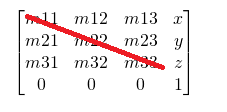Note that `m11`, `m22`, `m33` will be 1 in the identity CFrame obtained by `CFrame.new()`. However, there will be only 3 `1`'s in the identity CFrame, not 4. This is because `0, 0, 0, 1` technically isn’t supposed to exist in the matrix but does (so you can multiply CFrame together by obeying the rule mentioned above), so only the `1`'s in the diagonal line in the rotational component of the matrix (see the image above) are counted. Hopefully this makes sense, and this can be also seen in the code below:

``````local x,y,z, m11, m12, m13, m21, m22, m23, m31, m32, m33 = CFrame.new():GetComponents()
print(m11, m22, m33) --> 1, 1, 1
print(m12, m13, m21, m23, m31, m32) --> 0, 0, 0, 0, 0, 0
print(x, y, z) --> 0, 0, 0
``````

The following unit vectors are the “directional” vectors which can be accessed through a CFrame only, these unit vectors are derived from the rotational component of a CFrame.

• RightVector → The right direction of a CFrame.
• UpVector → The up direction of a CFrame.
• LookVector → The forward direction of a CFrame.

When negating these vectors, you get the opposite direction.

## Unit Vectors

Unit vectors do come in some or probably a lot of cases when working with CFrames, so I’ve included them here as well. They are basically vectors always have a magnitude (length) of approximately 1. The vectors (`UpVector`, `LookVector`, `RightVector`) are also unit vectors as they also indeed have a magnitude of approximately 1.

To get the unit of a vector, simply index the `Unit` member of a vector:

``````local vector = Vector3.new(50, 50, 50)

print(vector.Unit) --> 0.57735025882721, 0.57735025882721, 0.57735025882721
print(vector.Unit.Magnitude) --> 0.99999988079071 (approx 1)
``````

#### A unit vector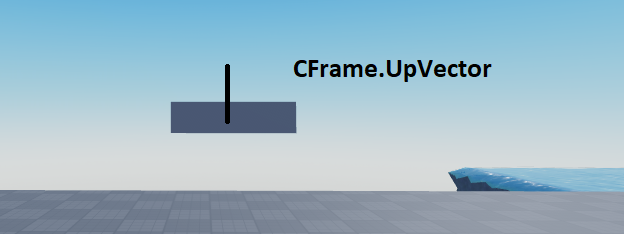#### A normal vector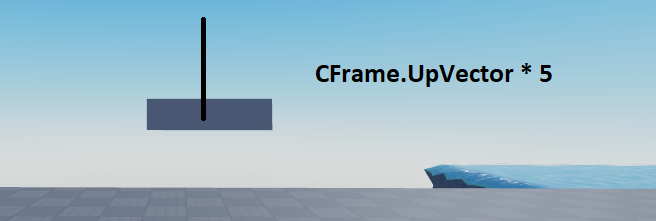# CFrame math

This section will cover CFrame math, along with the concept of relative positioning and rotating.

What If I were to told you that you can actually create a CFrame out of 12 numbers instead of 3.

``````local cf = CFrame.new(5, 5, 5, 1, 0, 0, 0, 1, 0, 0, 0, -1)
``````

The first 3 arguments make up the positional component of the CFrame, while the last 9 arguments make up the rotational component of the CFrame.

• `CFrame.XVector` is the vector in the first column in the rotational component, which means that `CFrame.RightVector` is the same as `CFrame.XVector`.

• `CFrame.ZVector` is the vector in the last column of the rotational component, which means that `CFrame.LookVector` is the same as `CFrame.ZVector`.

• `CFrame.YVector` is the vector in the middle column of the rotational component, which means that `CFrame.UpVector` is the same as `CFrame.YVector`.

CFrames and Vector data types are implemented in C++ and use floats internally, not doubles (64 bit floats). So each value stored in these data types take 32 bits, not 64 bits and hence these values are only precise up to 7 significant digits.

``````local vector = Vector3.new(0.1, 0.1, 0.1)

-- Float representation:
print(vector.X)  --> 0.10000000149012 (accurate up to 7 significant digits)

-- Double representation:
print(("%.17f"):format(0.1)) --> 0.10000000000000001 ( accurate up to 16 significant digits)
``````

### CFrame * CFrame

Multiplying 2 CFrames is involves matrix multiplication which is done internally. This is matrix multiplication is abstracted from the developer, so you don’t need to know this. Also, `CF1 * CF2` is the same as `CF1:ToWorldSpace(CF2)`.

Note that CFrame multiplication is not commutative, exceptions include multiplying a CFrame with it’s inverse, which will always return the identity CFrame! Multiplying a CFrame (suppose `CFrame1`) by another CFrame results in a newly created CFrame relative to `CFrame1`. In the picture below, the part is moved 4 studs relative to it self.

Similarly, if the part was rotated:

You can see that it moved relative to it self again because we can see that it moved relative to it self because it accounted for it’s orientation when moving. The `UpVector` of the part was rotated, and the part moved 4 studs respecting it’s rotation which in this case, was the `UpVector` or the “top” of the part.

### CFrame +/- Vector3

Adding / subtracting a vector from a CFrame will result in a CFrame which will be positioned relative to the origin because the vector will simply be added to the positional component of the CFrame.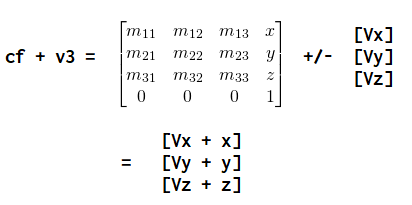Through this picture, we can see that the rotation of the CFrame is ignored. This clearly tells us why the resultant CFrame is positioned relative to the origin.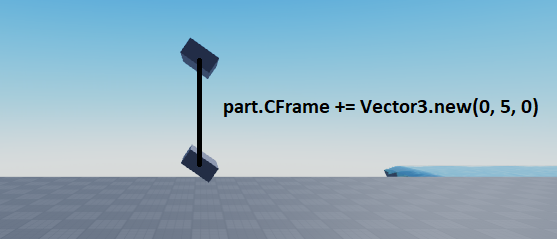As you can see, the part simply moved 5 studs relative to the origin instead of it’s own orientation, because it didn’t move relative to it’s up vector but rather the origin’s “up”.

### CFrame * Vector3

Multiplying a CFrame with a vector is the same as multiplying a CFrame with another CFrame, except that the former will yield a vector and the latter will yield a CFrame. We can see the multiplication of a vector with a CFrame: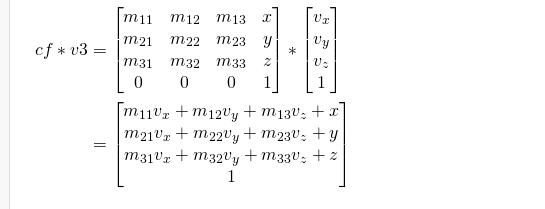It can be seen through this picture, that the rotation of the CFrame is taken into account, so it makes complete sense that the resultant vector is relative to the CFrame.

### Relative CFrame rotation

Rotating a CFrame is as straight forward as it can get, so this section won’t go in much detail. CFrame rotation involves the method `CFrame.Angles` (and some other methods but we’ll cover this method as it is the main one), which takes 3 values as it’s arguments and returns a rotated CFrame from those 3 values. Most of the times, you need to make sure that the values passed are in radians and not in degrees for expected rotation, however there are quite a lot of exceptions as well but that won’t matter for now.

Let’s create a part on the client which rotates a part relative to it self smoothly every frame, and with respect with how much time a frame takes.

``````local ANGLE_ROT = 80

local part = Instance.new("Part")
part.Anchored = true
part.Parent = workspace

game:GetService("RunService").RenderStepped:Connect(function(deltaTime)
part.CFrame *= CFrame.Angles(0, math.rad(ANGLE_ROT * deltaTime * 2), 0)
end)
``````

Additionally, a little more stupid math and we can create a rapidly stupidly rotating part lol:

``````local ANGLE_ROT = 120

local part = Instance.new("Part")
part.Anchored = true
part.Parent = workspace

game:GetService("RunService").RenderStepped:Connect(function(deltaTime)
local rotY = math.rad(ANGLE_ROT * deltaTime * 2)
part.CFrame *= CFrame.Angles(-rotY * math.sqrt(deltaTime), rotY, -math.deg(math.sqrt(rotY)))
end)
``````

## Closing

Thanks for reading this quick article on CFrames, you may want to check out this article on CFrame math operations only if you have properly grasped this article fairly as that article strongly assumes you are familiar with CFrame and vector math. Additionally, feel free to ask any questions you may have and good luck with your CFrame journey.

82 Likes

But great tutorial anyway! Really helpful!Oh ok then. Thanks for letting me know.

Tutorials is better I think, resources is more like links to stuff u made

I think you made a mistake here, you said back instead of up

3 Likes

Really awesome tutorial, explains a lot.

Good Job Bobo. You have made us proud

Amazing tutorial man! However on this

The rule actually is that 1 of their rows or columns must be the same. I am not sure of the 0,0,0,1 but it wouldn’t make much sense to treat the 1 as the scalar. Perhaps maybe it is the scale factor?

To add on this is a tiny bit petty but could you specify the inverse of a cframe is the additive inverse or in other words negative or positive inverse? Bringing each of its components to the power of -1 is what I thought at first when learning cframes

No, the rule is that the two numbers considered to be “inside” when the two orders of the matrices are adjoined must be the same for mulitplication to happen (i.e: going from left to right, the first matrix’s number of columns must be equal to the number of rows in the second matrix. Note that the order of a matrix is represented as “(# of rows) x (# of columns)”)

This also makes it easier to calculate the determinant of the matrix, which is in turned, used to calculate the inverse of the matrix (generally in math, but it’s easier with just the transpose of the rotation matrix since the vectors are orthogonal), since you’d most likely be looking for the row with the most 0’s, which is always the last row.

It is a scalar. The 1 is only there to preserve the identity matrix. An identity matrix is the matrix where all of the numbers in the leading diagonal are 1 and the rest 0. The identity matrix of a cframe (and generally for all 4x4 matrices) is: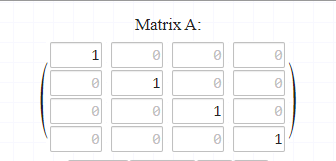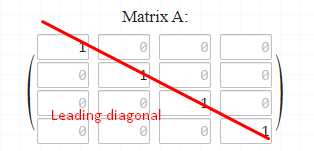It can be obtained from using a blank cframe, i.e: calling CFrame.new without any arguments: `CFrame.new()`

In some cases, negating the positional components and transposing the rotation matrix will give you the inverse, but in other cases, negating the positional components won’t give you the correct inverse. These cases that it would work in is when the cframe has default rotation. Mind you that the inverse of a matrix satisfies the equality of `A * A^-1 = I`, A being a matrix, A^-1 being matrix A’s inverse and I being the identity matrix

All in all, it’s a pretty good and comprehensive tutorial

1 Like

Nice job man! This is really good!
One thing I noticed however, in the picture of the Mouse.Hit lookvector, it’s rotation is actually determined by the camera’s lookvector instead of the character’s lookvector, but other than that it is phenominal!

`Mouse.Hit.LookVector` is relative to the camera and is based of `mouse.UnitRay`.

The CFrame of the mouse in 3D space is determined by `mouse.UnitRay`.

I’m not the best with drawings but in the picture, it is determined by the forward part of the mouse’s CFrame in 3D space so it shows the reader that `LookVector` is “forward”.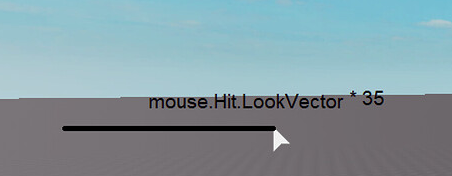This isn’t always true, Inverse is more than negation. What you told is only applicable when Right Vector, Up Vector and -Look Vector is `(1, 0, 0)`, `(0, 1, 0)` and `(0, 0, 1)` respectively.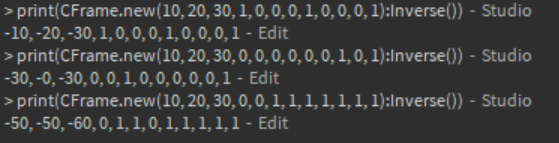I suggest you explain that in a bit more depth.

1 Like

(under CFrame.new())

Did you mean 3 positional and 9 rotational?